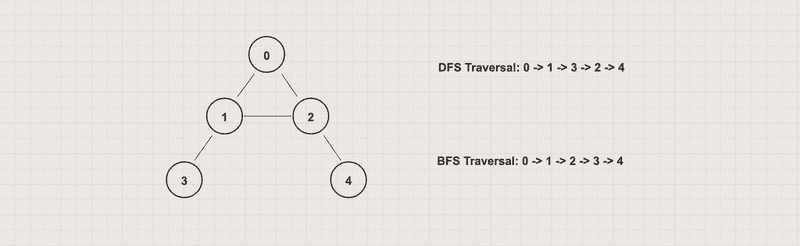# HelloKoding

Practical coding guides

# Breadth First Search Algorithms in Graph with Java Examples

In this article, you will learn to implement Breadth-First Search (BFS) algorithms in a graph by using Java with iterative approaches

Breadth-First Search (BFS) is an algorithm for traversing and searching for a graph/tree layer-wise. It starts at an arbitrary node and explores all of the neighbor nodes before moving to the next depth level

Let’s get started!

## Problem

• Give an undirected/directed graph G(V, E)
• Write a Breadth-First search algorithm to print out each vertex value exactly once

## ExampleFor the above graph with 0 as the starting vertex, assuming that the left edges are chosen before the right edges, the BFS traversal order will be 0 -> 1 -> 2 -> 3 -> 4

## Approach 1: Iterative

• Use an array to track visited nodes to avoid processing a node more than once
• Use a queue to track which nodes to visit next

BFSByIterative.java

``````package com.hellokoding.datastructure.graph;

import java.util.ArrayDeque;
import java.util.Deque;

public class BFSByIterative {
public static void bfsByIterative(GraphUndirectedByAdjacencyList g, int v) {
boolean[] visited = new boolean[g.getV()];
Deque<Integer> queue = new ArrayDeque<>();
queue.offer(v);

while (!queue.isEmpty()) {
v = queue.poll();

if (!visited[v]) {
visited[v] = true;
System.out.printf("%d ", v);

for (Integer w : g.getAdjacencyList().get(v)) {
queue.offer(w);
}
}
}
}

public static void main(String[] args) {

bfsByIterative(g, 0);
}
}
``````

`GraphUndirectedByAdjacencyList` is defined in Graph Data Structure

• Output
``0 1 2 3 4 ``
• Time complexity: O(V+E)
• Space complexity: O(V)

## Approach 2: Iterative with Color

• Use a color array to track vertex states. Each vertex can have 3 states marked by color

• White represents unvisited
• Gray represents a visit in progress
• Black represents visited
• Use a queue to track which nodes to visit next

BFSByIterativeWithColor.java

``````package com.hellokoding.datastructure.graph;

import java.util.ArrayDeque;
import java.util.Deque;

public class BFSByIterativeWithColor {
static final int WHITE = 0, GRAY = 1, BLACK = 2;

public static void bfsByIterativeWithColor(GraphUndirectedByAdjacencyList g, int v) {
int[] color = new int[g.getV()];
Deque<Integer> queue = new ArrayDeque<>();
queue.offer(v);

while (!queue.isEmpty()) {
v = queue.poll();

if (color[v] == WHITE) {
color[v] = GRAY;
System.out.printf("%d ", v);

for (Integer w : g.getAdjacencyList().get(v)) {
queue.offer(w);
}

color[v] = BLACK;
}
}
}

public static void main(String[] args) {

bfsByIterativeWithColor(g, 0);
}
}
``````

`GraphUndirectedByAdjacencyList` is defined in Graph Data Structure

• Output
``0 1 2 3 4``
• Time complexity: O(V+E)
• Space complexity: O(V)

## Applications

• Find shortest paths between vertices

The shortest path is a path between two vertices in a graph such that the total sum of the weights of the constituent edges is minimum

You can implement an algorithm to find the shortest path by using Breadth-First Search (BFS), Dijkstra, Bellman-Ford, and Floyd-Warshall algorithms